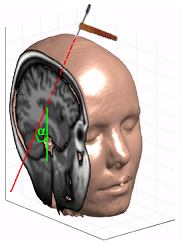# EndoScout Needle AnnotationThere are two different types of annotations:

• Perpendicular Annotation
• In-Plane Annotation

Which annotation type is used depends on the angle between the image slice and the needle. This angle (alpha) is shown in figure 1.

If alpha is between 60 and 90 degrees, the Perpendicular Annotaion is used. Otherwise, the In-Plane Annotaion is used.

Fig. 1 | Alpha is the angle between the slice and the needle.

# Perpendicular AnnotationThis annotation type is used when alpha is between 60 and 90 degrees.

A circle and a dot appear on the screen, as follows:

• The circle appears where the needle will enter the slice.
• The dot appears where the needle will exit the slice.

An example of this kind of annotation is shown in figure 2.

Fig. 2 | Perpendicular Annotation.

# In-Plane AnnotationThis annotation type is used when alpha is between 0 and 60 degrees.

A circle and a dashed line appear on the screen, as follows:

• The circle appears at the needle-tip location.
• The dashed line marks the projected path of the needle.
• A red dashed line represents the path before the slice.
• A yellow dashed line represents the path after the slice.
• A blue dashed line represents the path inside the slice.

An example of this kind of annotation is shown in figure 3.

Fig. 3 | In-Plane Annotation.